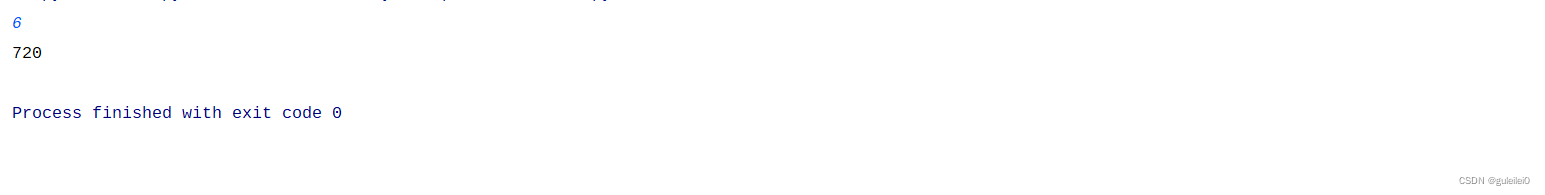### python计算n的阶乘

1.程序

``n = int(input())  def fact(n):    if n == 0 | n == 1:        return 1    elif n > 1:        return n * fact(n - 1)  print(fact(n))``

2.分析

3.实验结果